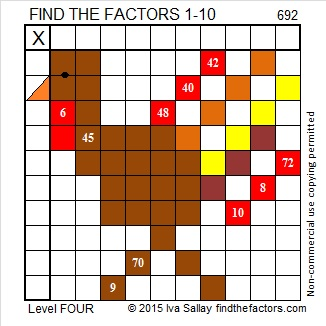# 692 Happy Thanksgiving!

• 692 is a composite number.
• Prime factorization: 692 = 2 x 2 x 173, which can be written 692 = (2^2) x 173
• The exponents in the prime factorization are 2 and 1. Adding one to each and multiplying we get (2 + 1)(1 + 1) = 3 x 2  = 6. Therefore 692 has exactly 6 factors.
• Factors of 692: 1, 2, 4, 173, 346, 692
• Factor pairs: 692 = 1 x 692, 2 x 346, or 4 x 173
• Taking the factor pair with the largest square number factor, we get √692 = (√4)(√173) = 2√173 ≈ 26.30589.Today is Thanksgiving in the United States. Regardless of where we live, there are reasons to be grateful today and every other day. Corrie ten Boom was grateful even when her circumstances were horrible. Here’s a Thanksgiving-themed puzzle to solve:Sometimes color in a puzzle is a distraction. Here’s the same puzzle minus the color:Print the puzzles or type the solution on this excel file: 10 Factors 2015-11-23

————————————————————————————

Here’s a little more about the number 692:

Because 173 is one of its factors, 692 is the hypotenuse of Pythagorean triple 208-660-692. What is the greatest common factor of those three numbers?

692 is a palindrome in 2 bases:

• 221122 BASE 3; note that 2(243) + 2(81) + 1(27) + 1(9) + 2(3) + 2(1) = 692
• 848 BASE 9; note that 8(81) + 4(9) + 8(1) = 692

————————————————————————————